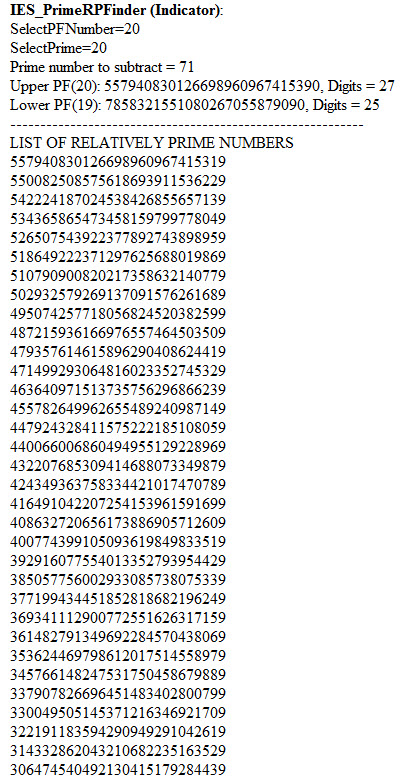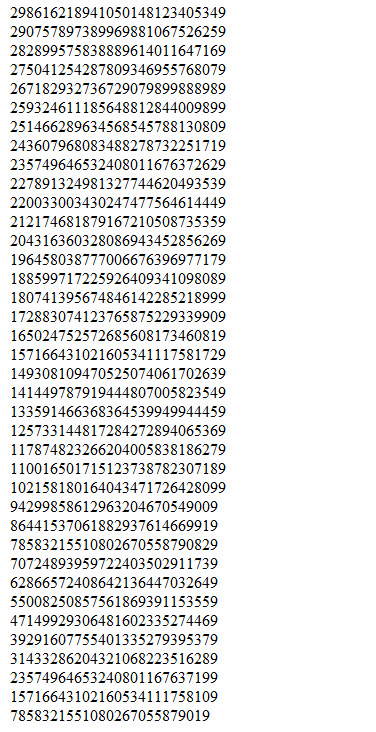Big Prime Number

# Searching for Prime Numbers

This is a demonstration of the method of searching for prime numbers using the matrix methodology. I have selected an upper prime factorial PF(20) and I chose the Key Seed number of 71. Subtracting 71 from PF(20) we get the first number in the list to the below. This is the 2nd largest number relatively prime to PF(20). Next we subtract the lower PF(19) number from the first number on the list and we get the second number on the list. We keep on subtracting the lower PF number until the last number on the list is less than the lower PF number. At that point we have found all the numbers relatively prime to the upper PF(20) that have a seed number that is equal to the lower PF minus 71 (not counting the lone number divisible by 71). You will see the rest of the list on the next page and you can verify that indeed it is exactly the lower PF – 71. Every one of these numbers is a possible prime number. Roughly 13% of the numbers in this list are prime.

Most of the numbers in the list have multiple factors. Some have only 2 factors and some are prime. Sorting out the prime from the non-prime is beyond the scope of this exercise but suffice it to say that there are tests that can be performed on these numbers to determine if they are prime. Similar sets of numbers can be computed by subtracting a larger prime number than 71 or any compound number whose only factors are prime numbers larger than or equal to 71. The only other columns of numbers would be based on the seed number 1 or PF(19) minus 1 in which case we would be adding PF(19) to those 2 numbers to get the rest of the numbers in the matrix.

Note that there are 71 numbers in this list. The last number is smaller than PF(19) so it is the seed for this column and is not part of the matrix. That demonstrates that the number of columns in the matrix is 1 less than the Key Seed number of 71.

Also notice that the last number is exactly equal to the PF(19) number minus the Key Seed. This is of course because I chose the Key Seed as the number to use in this exercise but it would have worked the same way no matter which of the seed numbers (larger than 71) I had chosen.

All of the code to perform computation on large numbers was generated by me in Easylanguage, a programming language for traders using TradeStation. Although there is probably a limit to how large a number can be computed, it is only limited by the size of the arrays that TradeStation can create. If you wish to obtain the complete library of code you can download it from my website at http://insideedge.net free of charge. However, you will not be able to use it unless you have TradeStation.